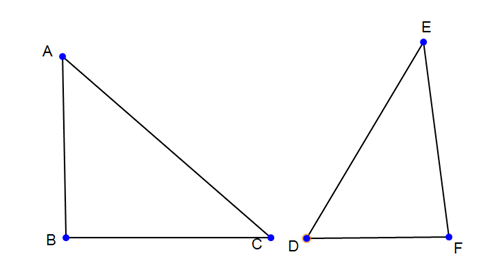##### KAPEC GEOTASK?
• The learning process is entertaining and exciting
• The solved tasks turn into coordinates
• The chance to follow your progress
• Along with your schoolmates and friends, go on an adventure, searching for hideouts
• E-mail:

# 1723.2. Triangle angle sum, straight angle cathetus, hypotenuse, Right-angled triangle similarity signs.

#### Give ∠ABC = ∠DFE = 90°!Is ∆ABC = ∆DFE, if AC = DE, AB=DF and BC=FE?

#### Next task: 838. Triangle angle sum, straight angle cathetus, hypotenuse, Right-angled triangle similarity signs.

Grade:
7
Subject
Geometry
Theme
Triangle angles sum. Right-angled triangle similarity signs.
Subtheme
Triangle angle sum, straight angle cathetus, hypotenuse, Right-angled triangle similarity signs.